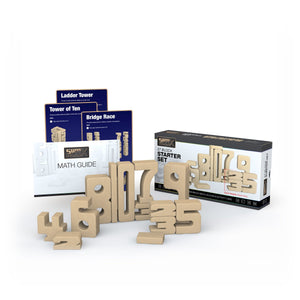Free UK shipping on orders over £40# SumBlox Starter Set

Regular price £74.99
Unit price  per
Tax included. Shipping calculated at checkout.

27-piece Starter bundle. In the set you get 10 number 1s, 2 of each number between 2 and 9, and 1 number 10. There are activity cards and a maths guide to Sumblox.

### For what age are SumBlox best suited?

SumBlox are great for children and adults of all ages but are best suited, academically, for children ages 2-12.

### What mathematical concepts can SumBlox teach?

Students can learn a wealth of information through play and hands-on exploration. These topics include:

##### Number Sense
• Number recognition
• One-to-one correspondence
• Compare numbers
• Subitizing
• Counting
• Cardinality
• Number sequence
• Hierarchical Inclusion
• Understanding our base-ten system
• Place value
• Measuring Lengths
• Reason abstractly and quantitatively
• Understand Equivalence
• Directly compare two objects with a measurable attribute in common
• Algebraic Thinking
• Describe measurable attributes of objects, such as length or weight
• Classify objects into given categories
• Solve Addition and Subtraction word problems using objects
• Compose and decompose numbers
• Skip counting
##### Multiplication / Division
• Define multiplication; emphasis on multiples
• Find factors of whole numbers and use commutative property of multiplication
• Classify whole numbers (greater than one) as prime or composite
• Draw conclusions about the factors of a whole number
• Use associative property of multiplication
• Use distributive property of multiplication